# Portfolio Characteristics

## Portfolio Expected Return

Thus far we have dealt with portfolios of at most two assets, with only one involving any risk. It is time to turn to the general relationship between the characteristics of a portfolio and the characteristics of its components.

Let there be n assets and s states of the world, with R an {n*s} matrix in which the element in row i and column j is the return (or value) of asset i in state of the world j. Here is an example with n=3 and s=4:

```             Good   Fair   Poor   Bad
Asset1      5      5      5      5
Asset2     10      8      6     -5
Asset3     25     12      2    -20
```

Let x be an {n*1} vector of asset holdings in a portfolio. For example:

```              x
Asset1    0.20
Asset2    0.30
Asset3    0.50
```

What will be the return of the portfolio in each of the states? This is easily computed. The {1*s} vector of portfolio returns in the states (rp) will be:

`   rp = x'*R`

Here:

```         Good    Fair    Poor    Bad
rp   16.50    9.40    3.80  -10.50
```

Now, let p be an {s*1} vector of the probabilities of the various states of the world. In this case:

```              p
Good    0.40
Fair    0.30
Poor    0.20
```

The expected return (or value) of the portfolio will be:

`    ep = rp*p`

In this case:

`    ep = 9.13`

It is useful to write the expression for expected return in terms of its fundamental components:

`     ep = x'*R*p `

The product of the three terms can be computed in either of two ways. Above, we computed x'*R, then multiplied the result by p. Alternatively, we could have multiplied x' by the result obtained by multiplying R times p:

`     ep = x'*(R*p) `

The parenthesized expression is an {n*1} vector in which each element is the expected return (or value ) of one of the n securities. Let e be this vector:

`     e = R*p`

Here:

```              e
Asset1    5.00
Asset2    7.10
Asset3   12.00
```

Using these results we may write:

`     ep = x'*e`

That is, the expected return (or value) of a portfolio is equal to the product of the vector of its asset holdings and the vector of asset expected returns (or values). This is the case whether the returns are discrete, as in this derivation, or continuous (that is, drawn from continuous distributions).

The units utilized for the values in vectors x and e will depend on the application. In some cases, physical units (e.g. shares) may be appropriate for x; in others, values (e.g. dollars); and in yet others, proportions of total value. Whatever the units selected, to find the end-of-period value of a portfolio, the end-of-period values per unit of exposure should be placed in vector e and the number of units of each asset held placed in vector x. To find the expected return (or value-relative) for a portfolio, multiply the expected returns (or value-relatives) in vector e by the exposures to the assets in vector x.

Whatever the application, the relationship between the expected outcome of a portfolio and the expected outcomes for its components is relatively simple and intuitive. For example, the expected return on a portfolio is a weighted average of the expected returns on its components, with the proportionate values used as weights. Since the relationship is linear, the marginal effect on portfolio expected return of a small change in the exposure to a single component will equal its expected outcome:

`   d(ep)/d(x(j)) = e(j)`

If the expected outcome were the only relevant characteristic of a portfolio, it would be easy to make investment decisions. But risk is also relevant. And, as we will see, its determination presents a more substantial challenge.

## Portfolio Risk

For present purposes we will use as a measure of portfolio risk the standard deviation of the distribution of its one-period return or the square of this value, the variance of returns.

By definition, the variance of a portfolio's return is the expected value of the squared deviation of the actual return from the portfolio's expected return. It depends, in turn, on the possible asset returns (R), the probability distribution across states of the world (p) and the portfolio's composition (x). The relationship is, however, somewhat complex.

To begin it is useful to create a matrix of deviations of security returns from their expectations. This can be accomplished by subtracting from each security return the corresponding expectation:

`   d = R - e*ones(1,s)`

The result (d) shows the deviation (surprise) associated with each security in each of the states of the world. Here:

```             Good    Fair    Poor    Bad
Asset1    0.00    0.00    0.00    0.00
Asset2    2.90    0.90   -1.10  -12.10
Asset3   13.00    0.00  -10.00  -32.00
```

The deviation (surprise) associated with the portfolio in each of the states of the world can be obtained by multiplying the transpose of the composition vector times the asset deviation matrix:

`    dp = x'*d`

In this case:

```         Good    Fair    Poor    Bad
dp    7.37    0.27   -5.33  -19.63
```

To determine the variance of the portfolio, we wish to take a probability-weighted sum of the squared deviations. A simple way to do so uses the dot-product operation, in which elements are treated one by one:

`   vp = sum(p'.*(dp.^2))`

However, there is a more elegant and (as will be seen) far more useful way to do the computation. First, we create a {s*s} matrix with the state probabilities on the main diagonal and zeros elsewhere. This can be done in one statement:

`   P = diag(p);`

In this case, P will be:

```           Good    Fair    Poor    Bad
Good    0.40    0.00    0.00    0.00
Fair    0.00    0.30    0.00    0.00
Poor    0.00    0.00    0.20    0.00
```

The variance of the portfolio is then given by a more conventional matrix expression:

`   vp = dp*P*dp'`

For our portfolio:

`   vp =  65.9641`

and

```   sdp = sqrt(vp)
= 8.1218
```

To see why the latter procedure for computing variance is more useful, we substitute the vectors used to compute dp:

`   vp = (x'*d)*P*(x'*d)'`

There is an easier way to write the last portion. Remember that the transpose operation turns a matrix on its side. From this it follows that:

`   (a*b)' = b'*a'`

For example, let a be a {ra*c} matrix and b a {c*rb} matrix. Then (a*b) is {ra*rb} and (a*b)' is {rb*ra}. Now consider the expression to the right of the equal sign. The first term (b') is of dimension {rb*c}, while the second is of dimension {c*ra}. Their product will thus be of dimension {rb*ra}. Since each element will represent the sum of the same set of products as in the result produced by the expression on the left, the resulting matrices will in fact be the same.

We can use this result to note that:

`   (x'*d)' = d'*x''`

But two transpose operations will simply turn a matrix on its side, then turn it back, giving the original matrix. Therefore:

`   (x'*d)' = d'*x`

And the expression for portfolio variance can be written as:

`   vp = (x'*d)*P*(d'*x)`

Of course the multiplications can be performed in any desired order. For example:

`   vp = x'*(d*P*d')*x`

The parenthesized term is of great importance in portfolio analysis - - enough to warrant its own section in this exposition.

## Covariance

The matrix described in the previous section is termed the covariance matrix for the assets in question. Each of its elements is said to measure the covariance between the corresponding assets. Using C to represent the covariance matrix:

`   C = d*P*d'`

In this example:

```           Asset1  Asset2  Asset3
Asset1    0.00    0.00    0.00
Asset2    0.00   18.49   56.00
Asset3    0.00   56.00  190.00
```

The variance of a portfolio depends on the portfolio's composition (x) and the covariance matrix for the assets in question:

`   vp = x'*C*x`

which of course gives the same value found earlier (65.9641).

Well and good. But what do the covariance numbers mean? How are we to interpret the fact that the covariance of Asset2 with Asset3 is 56.00, while that of Asset3 with itself is 190.00, and so on?

Examination of the matrices involved in the computation of C provides the answer. Recall that C=d*P*d'. Consider the covariance of Asset2 and Asset3. It uses the information in row 2 of matrix d and that in column 3 of matrix d' (the latter is, of course, also in row 3 of matrix d). It also uses the vector of probabilities along the diagonal of matrix P. The net result, written in a slightly casual notation is that:

`    C(2,3) = sum(d(2,s)*p'(s)*d(3,s))`

where the sum is taken over the states of the world.

As this expression shows, the covariance between two assets is a probability-weighted sum of the product of their deviations. To verify this we can adapt the expression above to make it legal in MATLAB:

`   c23 =   sum(d(2,:).*p'.*d(3,:))`

The answer is 56.00, precisely equal to the value in the second row and third column of the covariance matrix.

Put in terms of prospective results: the covariance between two assets is the expected value of the product of their deviations from their respective expected values. It immediately follows that the covariance of asset i with asset j is the same as the covariance of asset j with asset i. Thus the matrix is symmetric around its main diagonal -- note that the value in row 2, column 3 is the same as that in row 3, column 2. It also follows from the expression for covariance that the covariance of an asset with itself is its variance. The asset variances thus lie on the main diagonal of the covariance matrix. In this case:

`   va = diag(C)`

Here:

```               va
Asset1    0.00
Asset2   18.49
Asset3  190.00
```

The asset standard deviations are of course the square roots of these numbers:

`   sda = sqrt(diag(C))`

In this case:

```              sda
Asset1    0.00
Asset2    4.30
Asset3   13.78
```

Note that the first asset's return is certain. Hence its variance and standard deviation are zero. The second asset is risky, with a standard deviation of 4.30. The third is considerably more risky, with a standard deviation of 13.78.

Since the covariance matrix includes asset variances along the main diagonal, the entire matrix is sometimes termed a variance-covariance matrix. For brevity we will use the simpler term covariance matrix, but it should be remembered that the diagonal elements are both covariances and variances.

For the special case in which the probability of each state is the same, it is possible to compute the covariance matrix more simply using the standard MATLAB function cov. However, the function assumes that the inputs represent a sample of observations drawn from a larger population and hence adjusts the values in the matrix upwards to offset the bias associated with measuring deviations from a fitted mean. In effect, each value produced by the MATLAB function cov will equal the one given by our formulas times (s/(s-1)), where is the number of states (observations).

To use the cov function, simply provide the matrix of observations, with each row representing a different observation (state) and each column a different asset class. For example, if the returns in our {n*s} matrix R were historic observations and we were willing to assume that they were equally probable we could compute:

`   C = cov(R')`

which would give:

```           Asset1  Asset2  Asset3
Asset1    0.00    0.00    0.00
Asset2    0.00   44.92  122.58
Asset3    0.00  122.58  360.92
```

These values are, of course, quite different from those found earlier, due to both the assumption of equal probabilities and the correction for bias.

## Correlation

It is relatively easy to find a meaning for the elements on the main diagonal of the covariance matrix. But what of the remaining ones? How can one interpret the fact that the covariance of Asset2 with Asset3 is 56.00?

The solution is to scale each covariance by the product of the standard deviations of the associated assets. The result is the correlation coefficient for the two assets, usually denoted by the Greek letter rho:

`   rho(i,j) = C(i,j)/(sda(i)*sda(j))`

The matrix of correlation coefficients is termed (unimaginatively) the correlation matrix. We denote it Corr. To compute it, we compute a matrix containing the products of the asset standard deviations:

` sda*sda':`
```           Asset1  Asset2  Asset3
Asset1    0.00    0.00    0.00
Asset2    0.00   18.49   59.27
Asset3    0.00   59.27  190.00
```

We need to divide each element in the covariance matrix by the corresponding element in this matrix. This can be done in one equation:

`   Corr = C./(sda*sda')`

Giving:

```           Asset1  Asset2  Asset3
Asset1     NaN     NaN     NaN
Asset2     NaN    1.00    0.94
Asset3     NaN    0.94    1.00
```

Notice that the elements associated with asset pairs in which one of the assets is riskless are NaN (not a number), since they involve an attempt to divide zero (the covariance) by zero(the product of two standard deviations, one of which is zero).

While the correlation of two assets, one of which is riskless, is not really a number, it sometimes proves helpful to set it to zero. This can be accomplished by adjusting the matrix of the cross-products of the standard deviations to have ones in the cells for which the true value is zero. A simple way to do this is to add to the original matrix a matrix with 1.0 in such positions. Since "true" is represented in MATLAB as 1.0, a single matrix expression does the job. Here is a set of statements that accomplishes the objective:

```   z = sda*sda';
z = z + (z==0);
CC = C./z;
```

where CC is the desired correlation matrix. In this case:

```           Asset1  Asset2  Asset3
Asset1    0.00    0.00    0.00
Asset2    0.00    1.00    0.94
Asset3    0.00    0.94    1.00
```

In most cases, the covariance matrix is known, and the correlation matrix derived from it as an aid in interpretation. However, there are cases in which standard deviations and correlations are estimated first, and the covariance matrix derived from those estimates. To do this, we simply reverse the terms in the definition of correlation. For the element in row i, column j:

`   C(i,j) = rho(i,j)*sda(i)*sda(j)`

And, for the entire matrix:

`   C =CC.*(sda*sda')`

Note that the adjusted matrix CC was used in the latter computation to avoid NaN values in the cells associated with the riskless asset.

## Interpreting Correlation Coefficients

Asset covariances are the main ingredients for computing portfolio risks. But we have shown that standard deviations are much easier to interpret than are asset variances. Similarly, correlations often prove more useful for communicating relationships than do covariances.

Correlation coefficients measure the extent of the association between two variables. Each such coefficient must lie between -1 and +1, inclusive. A positive coefficient indicates a positive association: a greater-than-expected outcome for one variable is likely to be associated with a greater- than-expected outcome for the other while a smaller-than-expected outcome for one is likely to be associated with a smaller-than-expected outcome for the other. A negative coefficient indicates a negative association: a greater-than-expected outcome for one variable is likely to be associated with a smaller-than-expected outcome for the other while a smaller-than- expected outcome for one is likely to be associated with a greater-than-expected outcome for the other.

The figures below provide examples. In each case the probabilities of the points shown are assumed to be equal.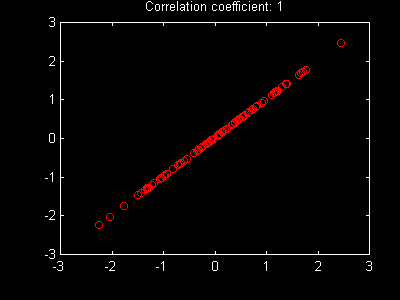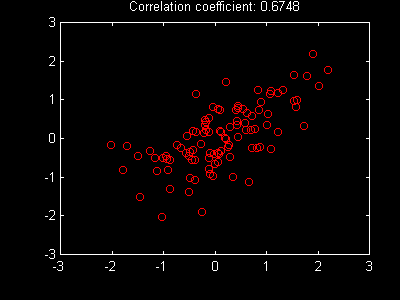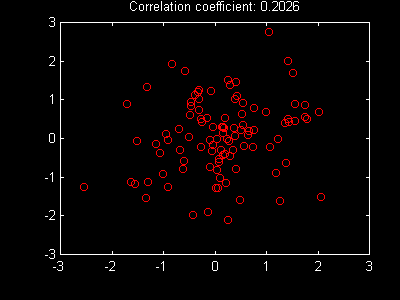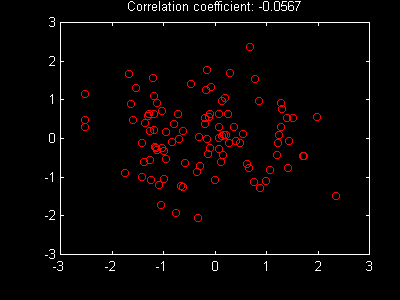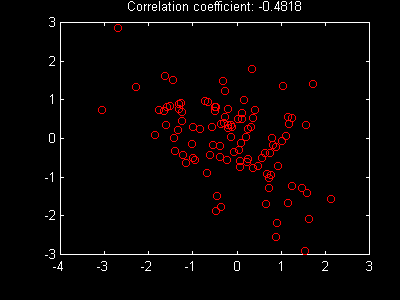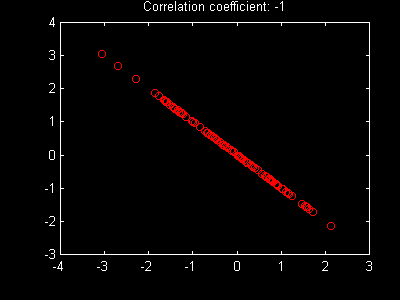In the above examples the variables are roughly jointly normally distributed with means of zero and standard deviations of 1.0 -- roughly, because each of the 100 points is drawn from such a joint distribution so the (sample) distribution of the actual results departs somewhat from the underlying (population) distribution.

Note that in the case of perfect positive correlation (+1.0), the points fall precisely along an upward- sloping straight line. In this case it has a slope of approximately 45 degrees due to the nature of the variables. In general, the line may have a greater or smaller slope. Nonetheless, a necessary and sufficient condition for perfect positive correlation is that all possible outcomes plot on an upward-sloping straight line.

In the case of perfect negative correlation the plot has the opposite characteristic. All points will plot on a downward-sloping straight line. Here too, the slope will depend on the magnitudes of the variables, but the line will be downward-sloping in any event.

As the figures show, in the case of less-than-perfect positive correlation (between 0 and +1.0), the points will tend to follow an upward-sloping line, but will deviate from it. The closer the correlation coefficient is to zero, the greater will be such deviations and the more difficult it will be to see any positive relationship. In the case of less-than-perfect negative correlation (between 0 and -1), the points will tend to follow a downward-sloping line. Here too, the closer the correlation coefficient is to zero, the greater will be the deviations and the more obscure the relationship.

If the correlation coefficient is zero, the best linear approximation of the relationship will be a flat line. This does not preclude the possibility that there is a non-linear relationship between the variables. The figure below shows a case in which the correlation coefficient is zero, but knowledge of the value of the variable on the horizontal axis would help a great deal if one wished to predict the value of the variable on the vertical axis. In this case the variables are uncorrelated, but they are not independent.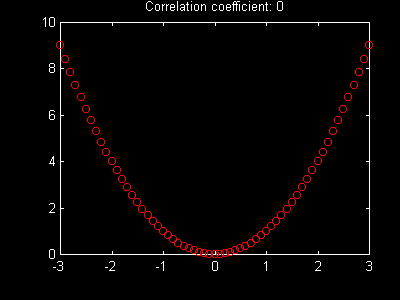In the special case in which probabilities are equal, one can use the MATLAB function corrcoef to compute a correlation matrix directly from an {n*s} matrix of values of n assets in s different states of the world, with each row representing a different state (observation) and each column a different asset. For example:

`   corrcoef(R')`

would give:

```           Asset1  Asset2  Asset3
Asset1     NaN     NaN     NaN
Asset2     NaN    1.00    0.96
Asset3     NaN    0.96    1.00
```

In this case the only source of the differences from our forward- looking estimates is the use of equal probabilities rather than the predicted probabilities. Since the correlation coefficient is the ratio of estimated variance to the product of two estimated standard deviations, any adjustment of the covariance matrix for sample bias cancels out, leaving the correlation coefficients unaffected.

## Exponentially Weighted Covariances

Analysts frequently utilize historic returns to estimate the covariances among future returns. If all returns, past and future, were drawn from a stable joint distribution, it would be desirable to use as many observations from the past as possible in order to maximize the accuracy of the resultant estimates of the true underlying process that will generate future returns. However, if the parameters of the distribution are likely to have changed over time, the situation is more difficult. One can utilize a great deal of data, much of which may be of limited relevance for the future. Alternatively, a small amount of recent data can be employed, with the attendant danger of substantial estimation errors. Which is better -- a great deal of possibly irrelevant data or too little relevant data?

There is no easy answer to the question. The optimal procedure ultimately will depend on the manner in which covariances evolve through time. Some Analysts approach the problem by limiting the historic data to, say, 60 monthly observations, with each observation assigned the same weight (probability). Others select only periods in which underlying conditions are assumed to have been similar to those existing at the present time (e.g. periods following recessions if a recession has recently been experienced). Yet others employ complex procedures in which covariances are assumed to be positively correlated but with a tendency to eventually revert to a long-run mean value. Here we focus on a simple procedure utilized in a number of asset allocation models that assumes that the future is more likely to be like the recent past than the distant past.

In an exponential weighting scheme each historic observation is assigned a multiple of the weight assigned to its predecessor. For example, observation t could be assigned a weight equal to 2^(t/h) divided by a constant (k), with the latter set so that the sum of all the weights equaled 1.0. In such a scheme h can be interpreted as an assumed half-life. To see why, consider the weights assigned to months t and t-h:

```   w(t)   = (2^(t/h))/k
w(t-h) = (2^((t-h)/h))/k
```

Thus:

`   w(t)/w(t-h) = (2^(t/h))/((2^t/h)/2) = 2`

Thus if month t is the most recent month and h=60, the observation 60 months ago will be assigned half as much weight as the most recent month.

The weight assigned to any month relative to that assigned to its predecessor will be:

`   (2^(t/h))/(2^((t-1)/h))`

which will equal 2^(1/h). Thus if a 60-month half life is utilized, each month's observation will be given a weight equal to 2^(1/60) or 1.0116 times that given the prior month (1.16% higher).

It is relatively straightforward to compute a set of such weights using MATLAB. Assume that there are T observations. The vector of dates (1,2,...T) is given by:

`   d = 1:1:T;`

The vector of 2^(t/h) values will be:

`   w = 2.^(d/h)`

where h is the desired half-life.

The weights can easily be normalized so that they sum to 1.0:

`   p = w/sum(w)`

We denote the result p since the weights will serve as probabilities. In a sense, the assumption is made that the probability is p(t) that next month's returns will equal those that occurred in month t.

Having selected a set of probabilities, we proceed as before to estimate expected returns, deviations and the covariance matrix:

```   e = R*p;
d = R - e*ones(1,T);
C = d*diag(p)*d';
```

## Function wcov

The library function wcov obviates the need to remember all these formulas. It takes as inputs a matrix of returns for n assets in s states (or from s historic time periods). For convenience, the return matrix can have assets in the rows and states (observations) in the columns or vice-versa. The function assumes (reasonably) that the number of states (observations) exceeds the number of assets and proceeds accordingly.

To cover more cases, the half-life parameter can be specified as zero, in which event the states (observations) are given equal weights.

The simplest way to utilize the function is as follows:

`   C = wcov(R,h)`

where R is the matrix of returns, h is the half-life and C is the resultant covariance matrix.

`   [C,sda]  = wcov(R,h)`

will also return sda as the vector of standard deviations.

The statement:

`   [C,sda,CC]  = wcov(R,h)`

will also return CC as the matrix of correlation coefficients, following the convention that the correlation coefficient is zero if the corresponding covariance is zero.

Finally, the statement:

`  [C,sda,CC,e] = wcov(R,h)`

will also return the expected returns, based on the assumption that future probabilities equal the weights computed from the assumed half-life.

## Weighted Statistics Worksheet

The Weighted Statistics Worksheet allows you to compute both equal-weighted and exponentially-weighted means, standard deviations and correlation coefficients. An example using returns for a set of Vanguard mutual funds from 1991 through 1995 provides a chance for you to experiment with different weighting schemes. Try a half-life of zero for equal weights, then compare the results with those obtained with other values (for example, 12, 24, .. 60). You might even wish to try a negative half-life to weight earlier observations more heavily than later ones.

You may also wish to copy and paste other return series into the weighted statistics worksheet so that you can calculate the resulting historic statistics.

## Portfolio Covariances

It is remarkably easy to determine the covariances between two portfolios. Recall the formula for computing the covariance of portfolio x:

```   vp = x'*C*x
```

where x is the vector with the portfolio composition and C is the covariance matrix for asset returns.

Now, let xa represent one portfolio and xb another. For example:

```              xa
Asset1    0.10
Asset2    0.50
Asset3    0.40
```
```              xb
Asset1    0.40
Asset2    0.10
Asset3    0.50
```

Assume that the covariance matrix (C) is:

```           Asset1  Asset2  Asset3
Asset1    0.00    0.00    0.00
Asset2    0.00   18.49   56.00
Asset3    0.00   56.00  190.00
```

The covariance between the two portfolios is given by:

`   cab = xa'*C*xb`

Which in this case equals 55.16.

The relationship can be extended to cover a case in which there are multiple portfolios. Let X be an {n*p} matrix containing information on the composition of p portfolios of n assets. For example:

```               xa      xb
Asset1    0.10    0.40
Asset2    0.50    0.10
Asset3    0.40    0.50
```

Then the covariance matrix for the portfolios is given by:

```   Cp = X'*C*X
```

Which gives:

```          xa      xb
xa    57.42   55.16
xb    55.16   53.28
```

Note that the elements on the main diagonal indicate the variances of the two portfolios, while the other elements equal their covariance.

## Asset Covariances with a Portfolio

It is straightforward to compute the covariance of each asset with a given portfolio. Recall the statement for the covariance of portfolio xa with portfolio xb:

`   cab = xa'*C*xb`

This can be computed in two operations:

`   cab = xa'*(C*xb)`

For example, with xb:

```   Asset1    0.40
Asset2    0.10
Asset3    0.50
```

and C:

```           Asset1  Asset2  Asset3
Asset1    0.00    0.00    0.00
Asset2    0.00   18.49   56.00
Asset3    0.00   56.00  190.00
```

then

`   cab = xa'*cp`

where cp = C*xb, or:

```               cp
Asset1    0.00
Asset2   29.85
Asset3  100.60
```

Now, assume that xa contains only the first asset:

```               xa
Asset1    1.00
Asset2    0.00
Asset3    0.00
```

Clearly, the covariance of xa with xb will equal the first value in vector cp (0.00).

If xa contained only the second asset, its covariance with xb would equal the second value in vector cp (29.85). And so on.

The conclusion is not hard to reach. Vector cp contains the covariances of the asset classes with portfolio xb.

More generally, if:

`   cp = C*x`

then cp(i) is the covariance of asset i with portfolio x.

Note that the covariance of an asset with a portfolio will be a weighted average of its covariances with all the assets (including itself), with the composition of the current portfolio used as weights.

## Marginal Risks

The risk of a portfolio is not a linear function of the vector of its components. Rather, the variance of a portfolio is a quadratic function of its composition. This thwarts the intuition of most Analysts and Investors. Indeed, the nature of risk may be the single most important argument for the use of quantitative analysis in investment management. Neither Investors nor Analysts can be blamed for this fact. Nor can Harry Markowitz. Nature made risk a quadratic function. Markowitz only discovered it.

Given this central fact of investment life, it follows that the impact on the risk of a portfolio of a small change in the amount invested in a particular asset is not simply a function of the risk of that asset. The impact will depend on the covariances of the asset with all the other assets currently in the portfolio and on the composition of the portfolio.

Consider a portfolio x and a "difference vector" d. We wish to determine the effect on portfolio variance of a switch from portfolio x to portfolio x+d.

The variance of x will be:

`   vx = x'*C*x`

While that of (x+d) will be:

`   vv = (x+d)'*C*(x+d)`

The latter can be expanded by noting first that (x+d)'=x'+d', giving:

`   vv = (x'+d')*C*(x+d)`

then by multiplying out all the terms:

`   vv = x'*C*x + x'*C*d + d'*C*x + d'*C*d`

Since the first term of the latter expression equals the variance of x, the change in variance is given by the sum of the last three terms:

`   dvp = x'*C*d + d'*C*x + d'*C*d`

The first two terms are the same. This follows from the facts that: (1) the transpose of a scalar is the same scalar, (2) the transpose of a product of matrices is the product of their transposes, taken in reverse order and (3) the covariance matrix C is symmetric, so that its transpose equals the original matrix. Given this, we may write:

`   dvp = 2*d'*C*x + d'*C*d`

We are interested here in the effect on variance of a small change in the holding of one asset. Thus d will contain only one small non-zero element. For example,if we wished to know the effect of a small change in the holdings of asset 2 we could set:

```                d
Asset1    0.00
Asset2    0.01
Asset3    0.00
```

Since the elements in d will be either zero or very small (very much less than 1.0), the final term in the earlier expression (d'*C*d) will be even smaller. Indeed, as d approaches zero, the one element in d'*C*d will approach zero considerably faster, since it involves the square of the non-zero element in d. For purposes of computing the marginal effect of a change we may ignore the final term, giving:

`   dvp = 2*d'*C*x`

or

`   dvp = d'*2*C*x`

From this it follows that d(vp)/d(x(j)) will equal the j'th row of 2*C*x. More generally, 2*C*x is the vector of marginal risks of the asset classes:

`   mr = 2*C*x`

with mr(j) indicating the change in portfolio variance per unit change in the amount invested in asset j.

Note finally, that C*x is the vector of the covariances of the assets with portfolio x, which we have denoted cp. Thus:

`   mr = 2*cp`

and two times the covariance of an asset with a portfolio indicates the marginal risk of that asset, given the composition of the portfolio. In our case:

```               mr
Asset1    0.00
Asset2   59.70
Asset3  201.20
```

Thus variance would not be affected by a small change in the holding of asset 1. It would increase at a rate of 59.70 per unit change in Asset 2 and at a rate of 201.20 per unit change in Asset 3. Of course these figures hold only approximately for finite changes in the assets, with the error greater, the larger the underlying change. Moreover, they assume that only one element in the portfolio is changed. If the assets represent zero investment strategies this may be feasible. If, however, they are true investments, at least one holding will have to be decreased for another to be increased. We will take these aspects into account in later discussions. For now it suffices to have determined the vector of derivatives of variance with respect to asset holdings.